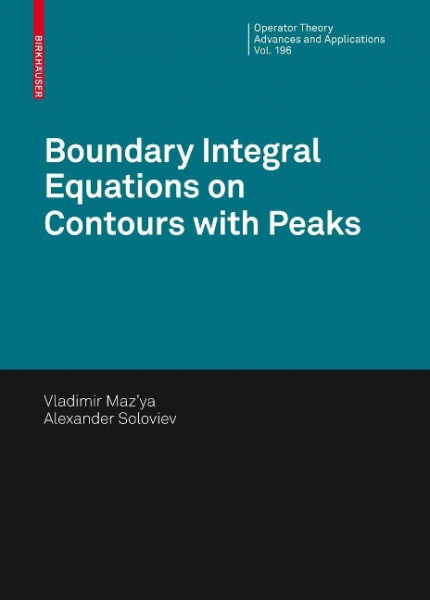Gebrauchte & Neue Bücher
Keine Versandkosten

# Boundary Integral Equations on Contours with PeaksGebrauchte Bücher kaufen

Information
Das Buch befindet sich in einem sehr guten, unbenutzten Zustand.
Information
Das Buch befindet sich in einem sehr guten, gelesenen Zustand. Die Seiten und der Einband sind intakt. Buchrücken/Ecken/Kanten können leichte Gebrauchsspuren aufweisen.
Information
Das Buch befindet sich in einem guten, gelesenen Zustand. Die Seiten und der Einband sind intakt. Buchrücken/Ecken/Kanten können Knicke/Gebrauchsspuren aufweisen.
Information
Das Buch befindet sich in einem lesbaren Zustand. Die Seiten und der Einband sind intakt, jedoch weisen Buchrücken/Ecken/Kanten starke Knicke/Gebrauchsspuren auf.

Neues Buch oder eBook (pdf) kaufen

Information
Neuware - verlagsfrische aktuelle Buchausgabe.
Zustand : Neu
149,99 €

inkl. MwSt. zzgl. Versandkosten

Lieferzeit 1 Werktag(e)

9783034601702

#### Die Studibuch PhilosophieWissen zu fairen Preisen, nachhaltig weitergeben.

#### Verwandte Sachgebiete

Produktdetails mehr
 Einband: Gebunden Seitenzahl: 356 Erschienen: 2009-11-19 Sprache: Englisch EAN: 9783034601702 ISBN: 3034601700 Reihe: Operator Theory: Advances and Applications 196 Verlag: Springer Basel AG Gewicht: 792 g
An equation of the form ??(x)? K(x,y)?(y)d?(y)= f(x),x?X, (1) X is called a linear integral... mehr
Produktinformationen "Boundary Integral Equations on Contours with Peaks"
An equation of the form ??(x)? K(x,y)?(y)d?(y)= f(x),x?X, (1) X is called a linear integral equation. Here (X,?)isaspacewith ?-?nite measure ? and ? is a complex parameter, K and f are given complex-valued functions. The function K is called the kernel and f is the right-hand side. The equation is of the ?rst kind if ? = 0 and of the second kind if ? = 0. Integral equations have attracted a lot of attention since 1877 when C. Neumann reduced the Dirichlet problem for the Laplace equation to an integral equation and solved the latter using the method of successive approximations. Pioneering results in application of integral equations in the theory of h- monic functions were obtained by H. Poincar¿ e, G. Robin, O. H¿ older, A.M. L- punov, V.A. Steklov, and I. Fredholm. Further development of the method of boundary integral equations is due to T. Carleman, G. Radon, G. Giraud, N.I. Muskhelishvili,S.G.Mikhlin,A.P.Calderon,A.Zygmundandothers. Aclassical application of integral equations for solving the Dirichlet and Neumann boundary value problems for the Laplace equation is as follows. Solutions of boundary value problemsaresoughtin the formof the doublelayerpotentialW? andofthe single layer potentialV?. In the case of the internal Dirichlet problem and the ext- nal Neumann problem, the densities of corresponding potentials obey the integral equation ???+W? = g (2) and ? ???+ V? = h (3) ?n respectively, where ?/?n is the derivative with respect to the outward normal to the contour. von Mazya, Vladimir
Weiterführende Links zu "Boundary Integral Equations on Contours with Peaks"
Bewertungen lesen, schreiben und diskutieren... mehr
Kundenbewertungen für "Boundary Integral Equations on Contours with Peaks"
Bewertung schreiben
Bewertungen werden nach Überprüfung freigeschaltet.

Die mit einem * markierten Felder sind Pflichtfelder.

Ich habe die Datenschutzbestimmungen zur Kenntnis genommen.